Author: Oscar Cronquist Article last updated on January 06, 2022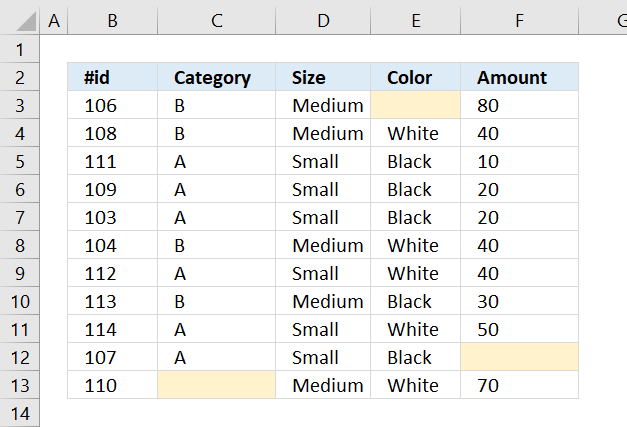## 1. Highlight empty cellsThe image above shows a data set containing a few random blanks. A conditional formatting formula highlights the empty cells yellow.

Conditional formatting formula:

=B3=""

### 1.1 Explaining conditional formatting formula

#### Step 1 - Specify relative cell reference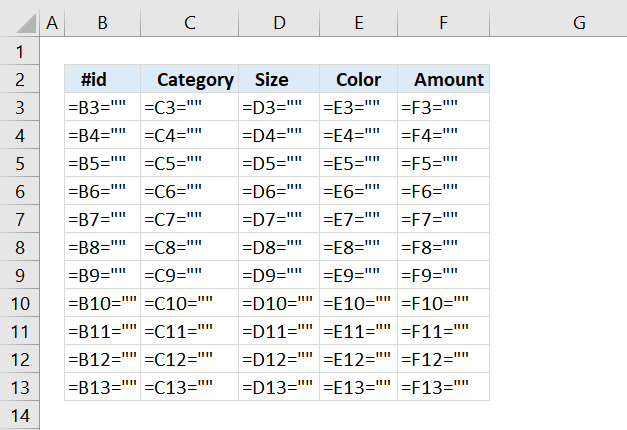The image above shows what the CF formula looks like in each cell, the actual cells do not contain this formula.

The cell range we want to highlight empty cells in is B3:F13 so the cell we must highlight first is the cell in the upper left corner in order to highlight the correct cells.

Cell B3 is a relative cell reference and changes in the cell range we want to format. If we started with B4 cell B3 will be highlighted if cell B4 is empty and we don't want that. We want to highlight the empty cell.

#### Step 2 - Create a logical expression

The equal sign is a logical operator and the result is a boolean value TRUE or FALSE.

B3=""

becomes

106=""

and returns FALSE. Cell B3 is not highlighted.

### 1.2 How to apply conditional formatting

The conditional formatting formula applied to cell range B3:F15 highlights all blank cells yellow. Here is how I did it:

1. Select cell range B3:F13.
2. Go to tab "Home" on the ribbon.
3. Press with mouse on the "Conditional Formatting" button.
4. Press with mouse on "New Rule..".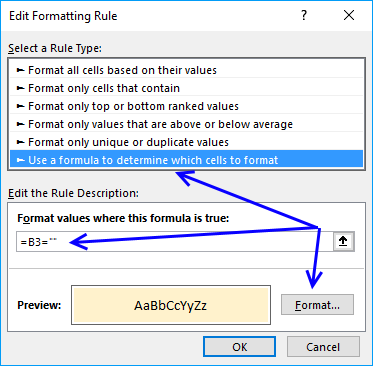5. Select "Use a formula to determine which cells to format".
6. Type =B3="" in field "Format values where this formula is true:".
7. Then press with left mouse button on "Format..." button.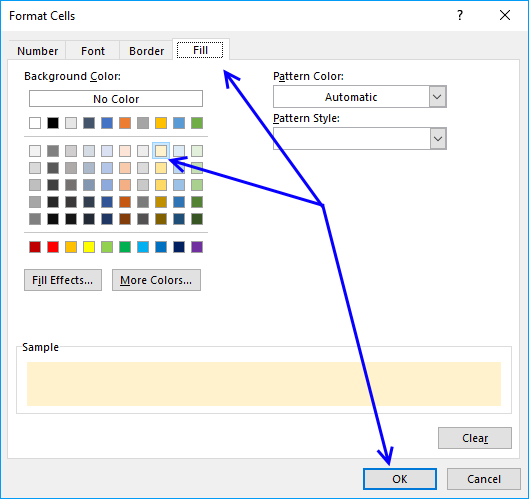8. Go to tab "Fill".
9. Pick a color.
10. Press with left mouse button on OK button.
11. Press with left mouse button on OK button to return to Excel.

### 1.3 Formulas returning nothing.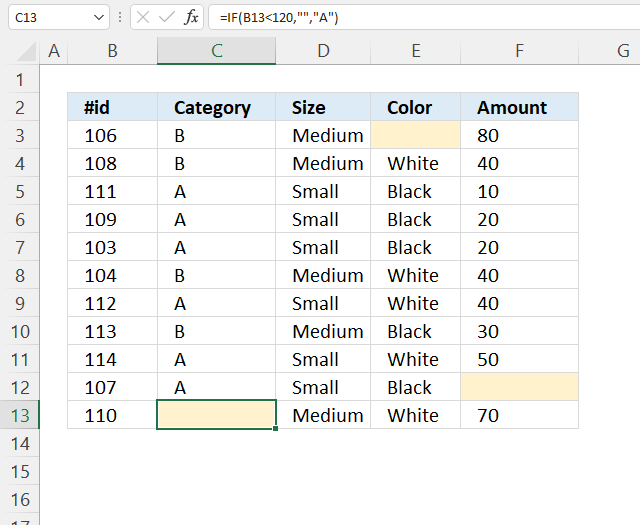Note, a cell is conditionally formatted if it contains a formula that returns nothing "". The cell is not highlighted if the formula returns a value.

The image above demonstrates a formula in cell C13 that returns nothing "", the cell is highlighted. Cell C13 is selected and the formula is displayed in the formula bar.

### 1.4 Cells with hidden values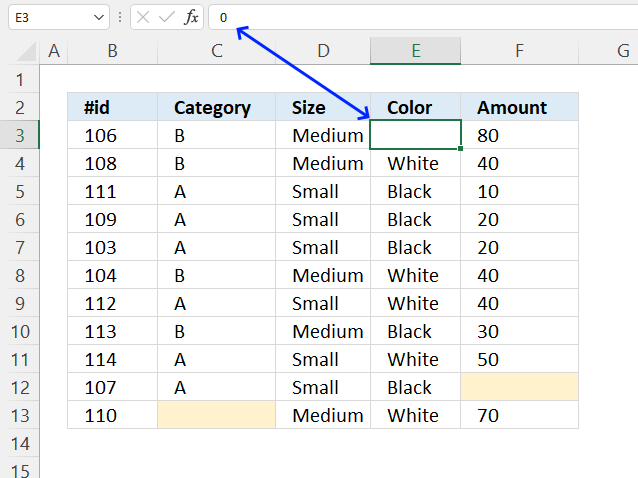The image above shows an empty cell E3 but it is not highlighted, cell E3 is actually not empty, it is only formatted to hide values.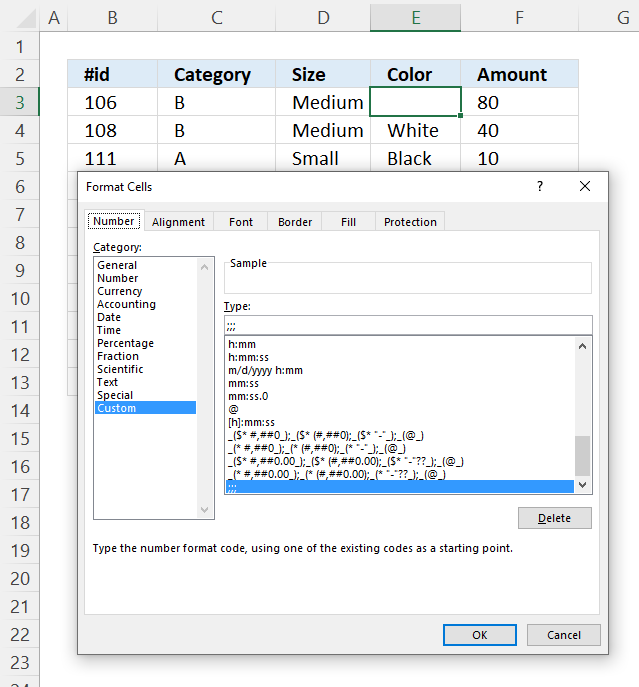Go to the "Format Cells" dialog box to inspect cells that should have been highlighted. The dialog box above shows cell E3 with a custom cell formatting. ;;; hides all values in a cell, select category: General to show the value again.

## 2. Highlight error cells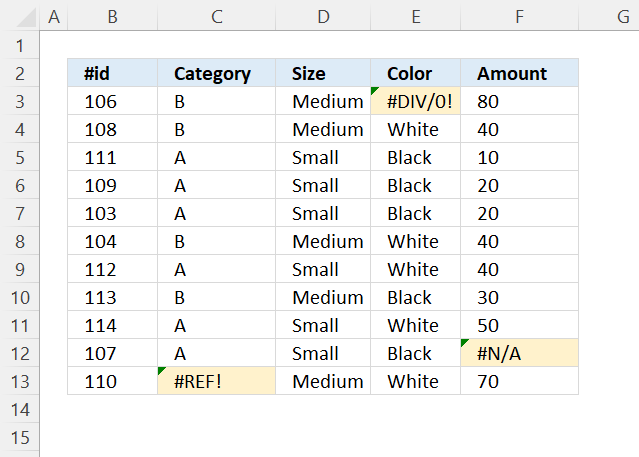The image above demonstrates a conditional formatting formula that highlights cells containing an error.

Conditional formatting formula:

=ISERROR(B3)

### 2.1 Explaining formula

#### Step 1 - Specify cell reference

The conditional formatting formula is applied to cell range B3:F13, the cell reference must point to the upper left cell which is cell B3 in this example.

B3 returns 106.

Cell reference B3 is a relative cell reference, it changes accordingly when the parser goes to the next cell. It is possible to use absolute cell references, however, this will not work in this example.

#### Step 2 - Identify error value

The ISERROR function returns TRUE if the value is an error value.

ISERROR(value)

ISERROR(B3)

becomes

ISERROR(106)

and returns FALSE. Cell B3 is not highlighted.

## Get Excel *.xlsx file

Highlight blank cells.xlsx